# Paper LBO - Step-by-Step Example

## Introduction

The dreaded paper LBO is a common obstacle during the private equity recruiting process.

Paper LBOs can be intimidating, in part, because they’re not something you do as a banker or a consultant. Maybe you make real LBOs, which are good preparation for private equity modeling tests, but paper LBOs are a different beast.

### The What

First, what is a paper LBO?

A paper LBO is a simplified LBO that can be completed with pen and paper quickly (ten minutes tops).

### The Why

Why does anyone bother with paper LBOs? What’s the point?

Paper LBOs are used during private equity interviews to test the following:

1. That you understand LBO mechanics well enough to perform the calculations with pen and paper – not using a well-trodden Excel template that has been passed down through generations of banking analysts
2. That you can do simple math quickly while under pressure - PE folks seem to think this is important ¯\(ツ)

Paper LBOs demonstrate that candidates can do private equity math on the spot, without Excel. Think back to the good ol’ days. Today’s titans of private equity didn’t even have Excel when they got started. They did some basic spreadsheet math, sure, but it wasn’t the complex analysis that you see today. The upper levels of private equity require suave professionals, who can do basic analysis in their head (or using an iPhone calculator) while golfing several beers deep. That’s a caricature, but you get the point.

So now we know what paper LBOs are and why folks bother. Let’s do one together.

## Paper LBO

Calculate the sponsor’s IRR and Multiple of Invested Capital (MoIC) using the information provided below:

### Transaction Assumptions

• 9.0x transaction multiple
• Cash-free, debt-free transaction
• 3.0x senior secured debt (4% interest rate, repayable immediately)
• 3.0x junior debt (8% interest rate, no early repayment)
• No transaction expenses

### LTM Financials

• 100mm Revenue
• 15mm EBITDA

### Projections

• 5% sales growth Y1 - Y3, followed by 3% sales growth
• Constant EBITDA margin
• 4mm CapEx Y1 - Y3, then 3mm CapEx per year
• 3mm depreciation & amortization (D&A) each year
• 2mm increase in net working capital (NWC) each year
• 30% tax rate

### Exit Assumptions

• Exit after 5 years at 9.5x LTM EBITDA
• Cash-free, debt-free transaction
• No transaction expenses

### Simplifying Assumptions

• Round all values to whole numbers (in millions). For example, if you calculate EBITDA using revenue (110mm) and the EBITDA margin (15%), the precise value is 16.5mm, but you can round it to 17mm. Adding decimal places is tedious and doesn’t demonstrate your understanding of LBO mechanics.
• Calculate interest expense based on the debt balance at the beginning of the given year.

## Try It Out

Below, we have the completed version along with a step-by-step guide. Before scrolling further, try building the paper LBO yourself. You should be able to complete it within 10 minutes. If it takes a little longer, that’s okay. Practice makes perfect.

## Completed Version

Here’s what our completed version looks like: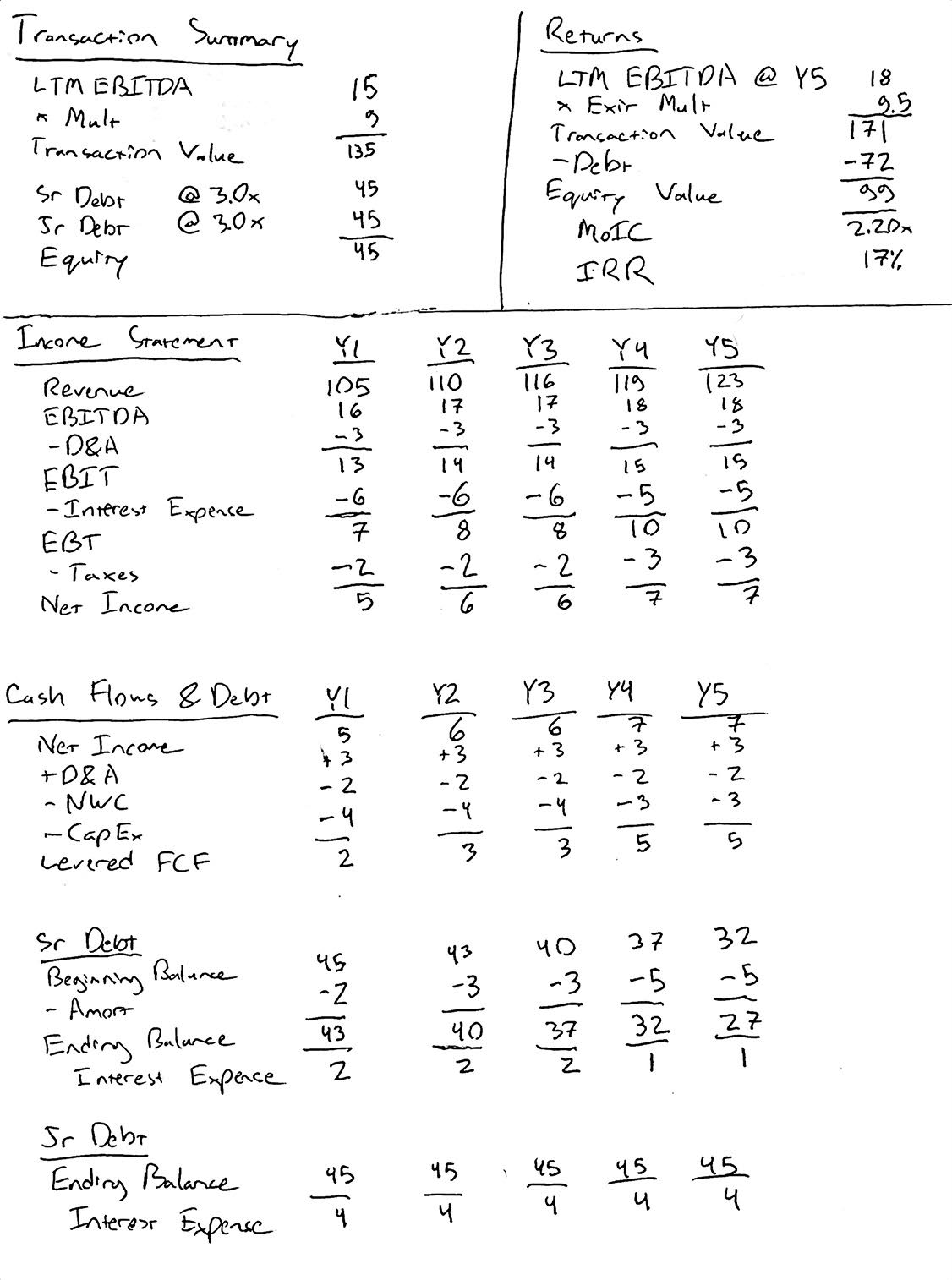We calculated the following sponsor returns:

• 2.20x MoIC
• 17% IRR

If you can’t read our handwriting, here’s the completed version in Excel.

## Step-by-Step Guide

### 1. Sketch It Out

This should feel somewhat familiar. Design your template on a sheet of paper. You’ll need the usual LBO sections:

• Transaction Summary
• Income Statement
• Cash Flow Statement
• Debt Schedule
• Returns Summary

Here’s what your “paper template” should look like: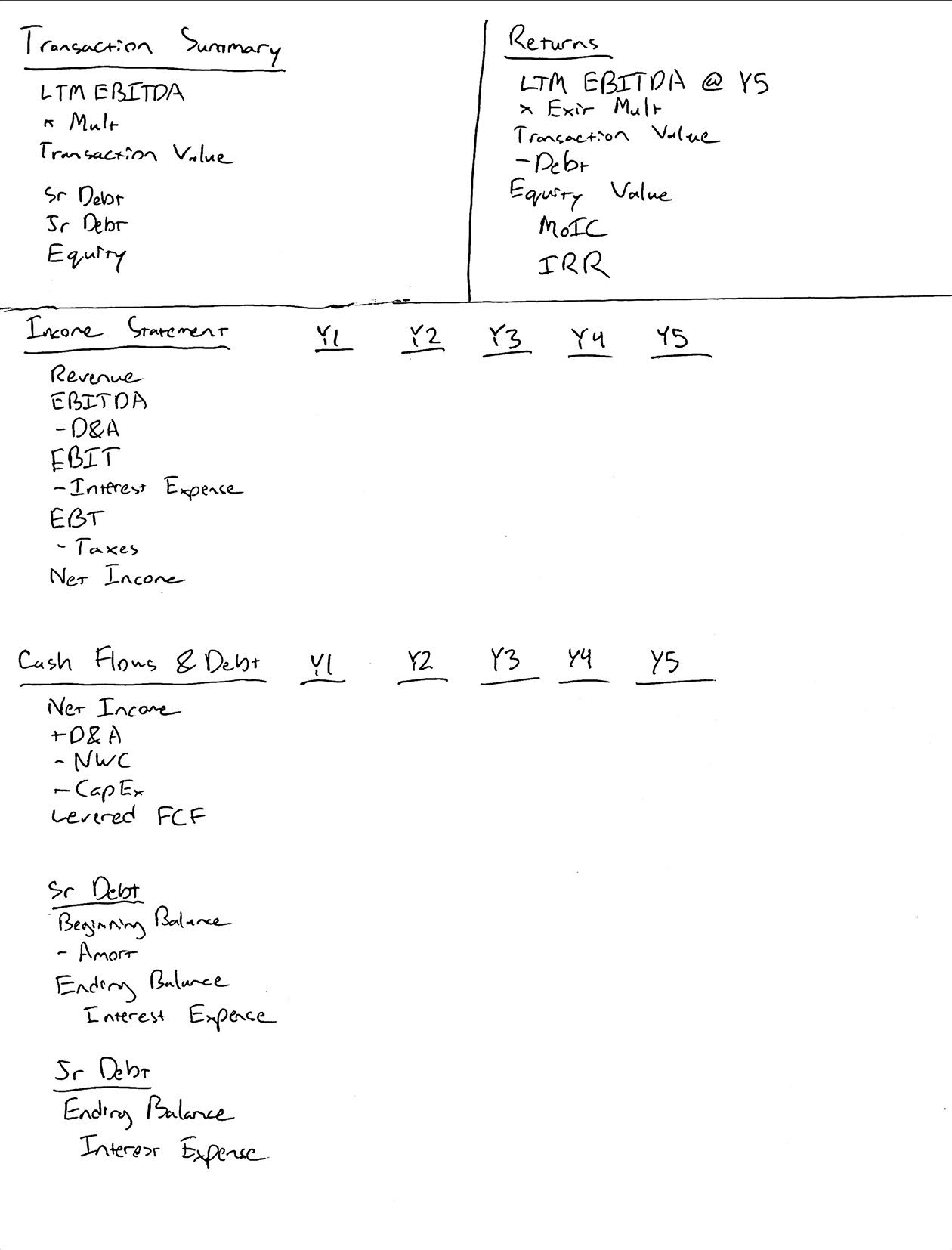### 2. Basic Financials

• Let’s fill out revenue, EBITDA, D&A, CapEx and change in NWC using our assumptions.
• D&A, CapEx and change in NWC are provided constants, so we only need to calculate revenue and EBITDA.
• The revenue values should be: 105, 110, 116, 119, 123
• The EBITDA values should be: 16, 17, 17, 18, 18

Remember: We’re rounding to whole numbers to make the calculations easier.

Here’s what our paper LBO looks like after this stage: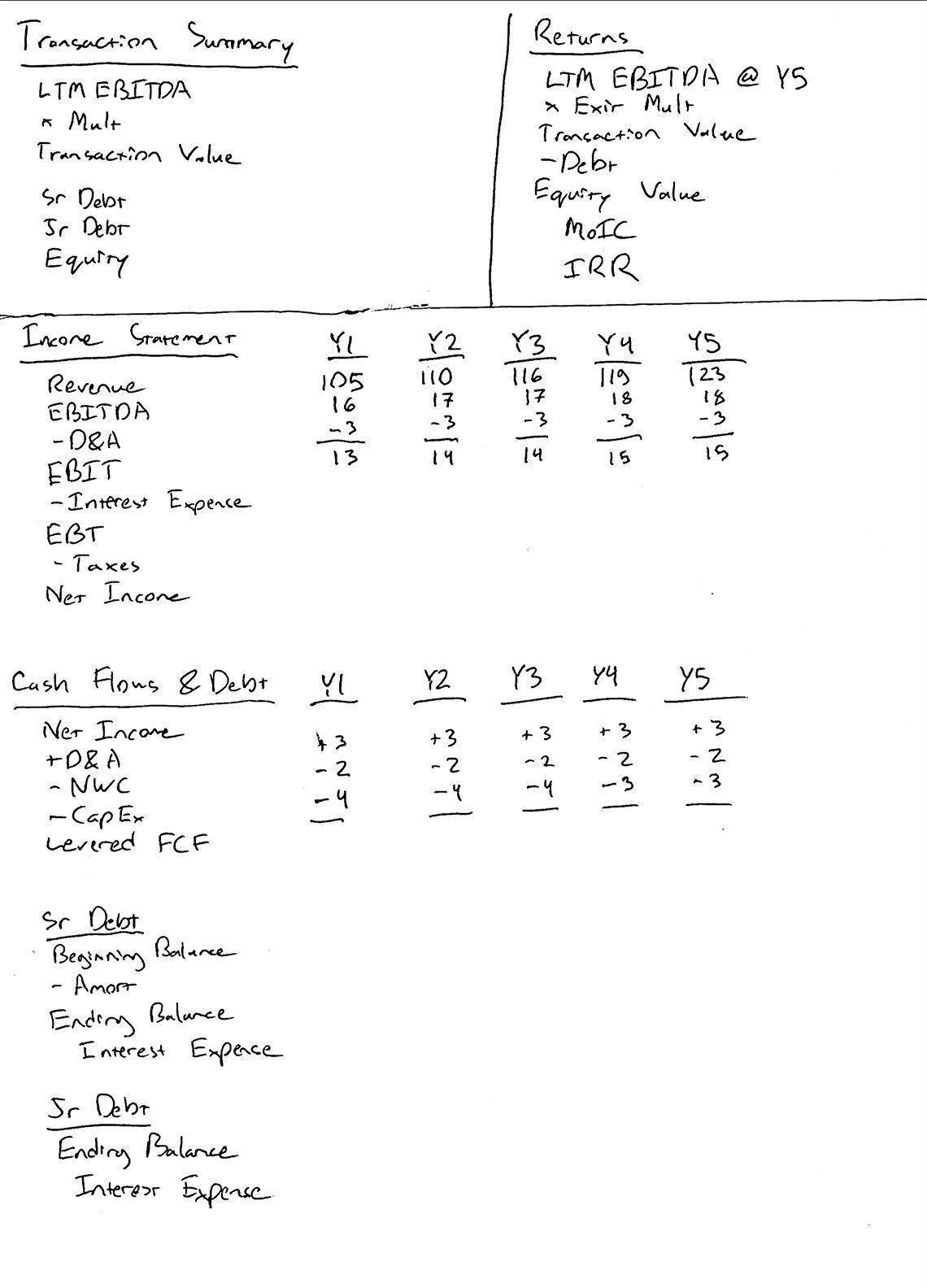### 3. Transaction Summary

Now let’s fill out the transaction summary.

• We know the transaction multiple and LTM EBITDA, so it’s easy to get the transaction value.
• Likewise, we know the LBO is financed with 3.0x senior debt and 3.0x junior debt. The remaining funds are provided by the sponsor (the private equity fund).

Here’s what the completed transaction summary looks like: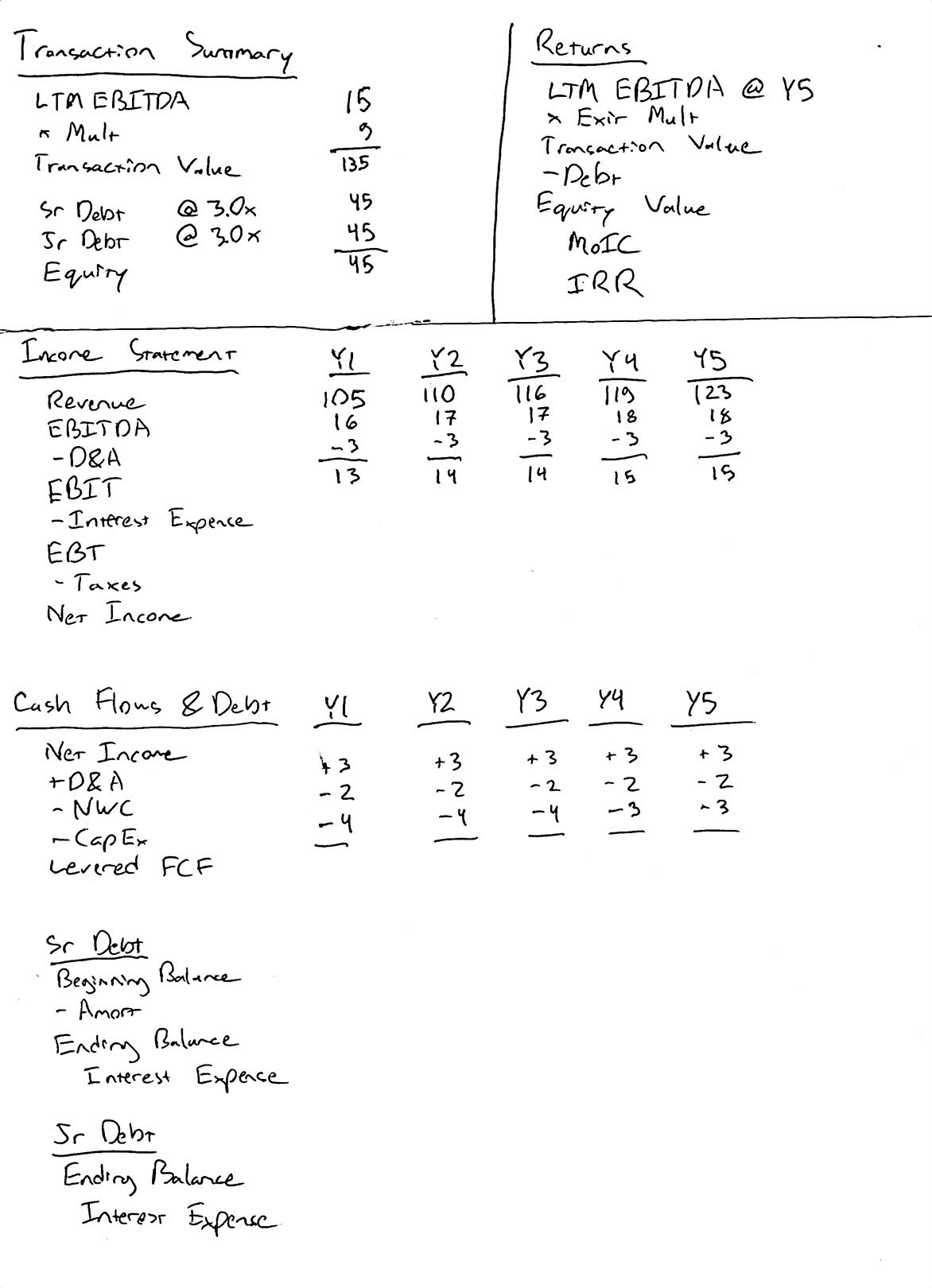### 4. Debt Schedule

In order to complete the income statement and the statement of cash flows, we need to calculate interest expense. Therefore, we need to begin filling out the debt schedule.

• We’re not paying down any of the junior debt, so the initial 45mm of junior debt will be held constant.
• We will, however, be paying down the senior debt.
• Since we’re calculating interest expense using the beginning debt balances each year, there’s no circularity. We can perform all of the calculations manually.

Let’s calculate the interest expense for year 1. We have 45mm of senior debt @ 4% and 45mm of junior debt @ 8%. Senior debt interest expense is 2mm (remember - rounding to whole numbers) and junior debt interest expense is 4mm.

Here’s what your paper LBO should look like so far: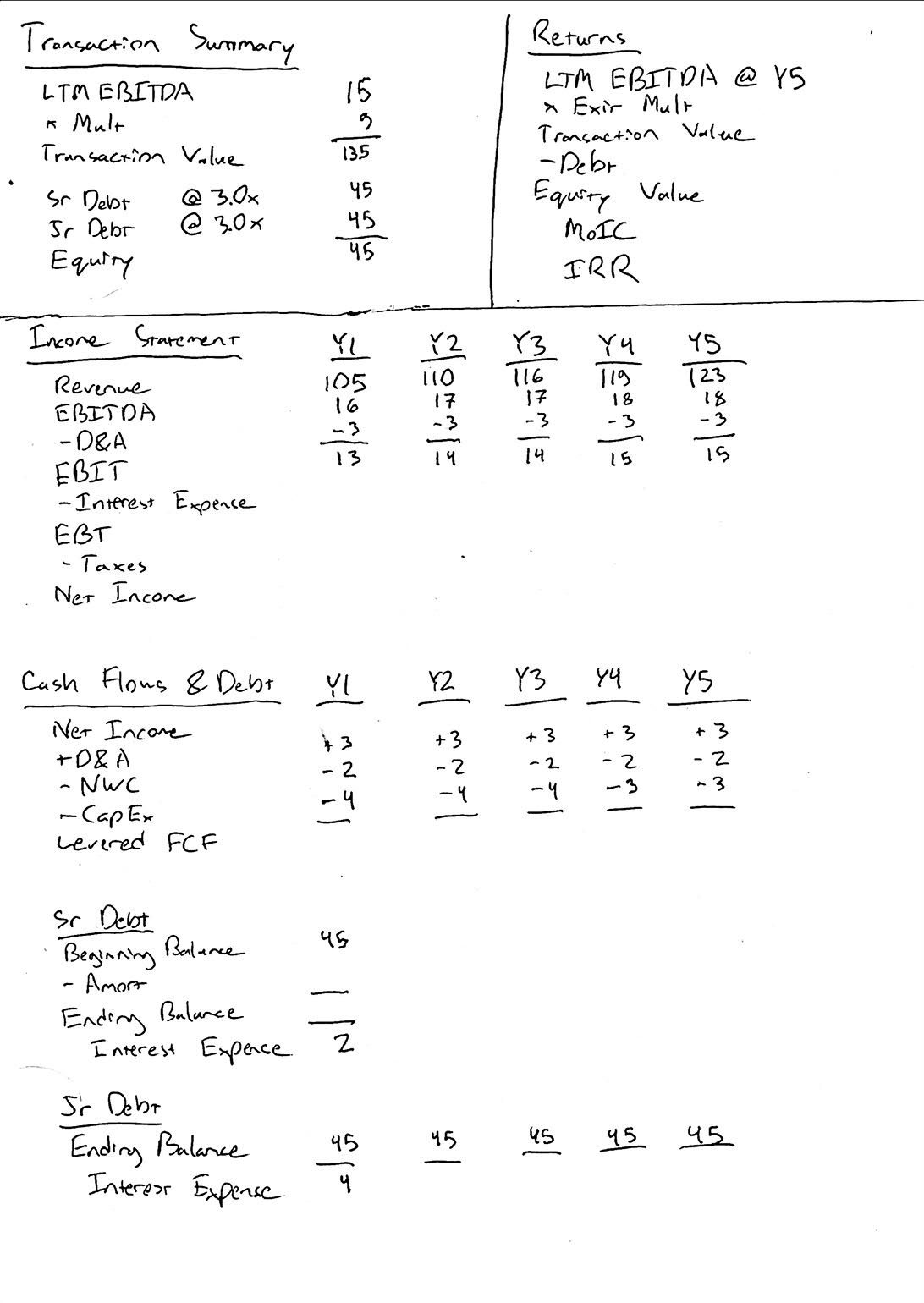### 5. Y1 Cash Flows

Since we have the Y1 interest expense (6mm total), we can complete the income statement and cash flows for Y1.

Here’s what your LBO should look like after this step: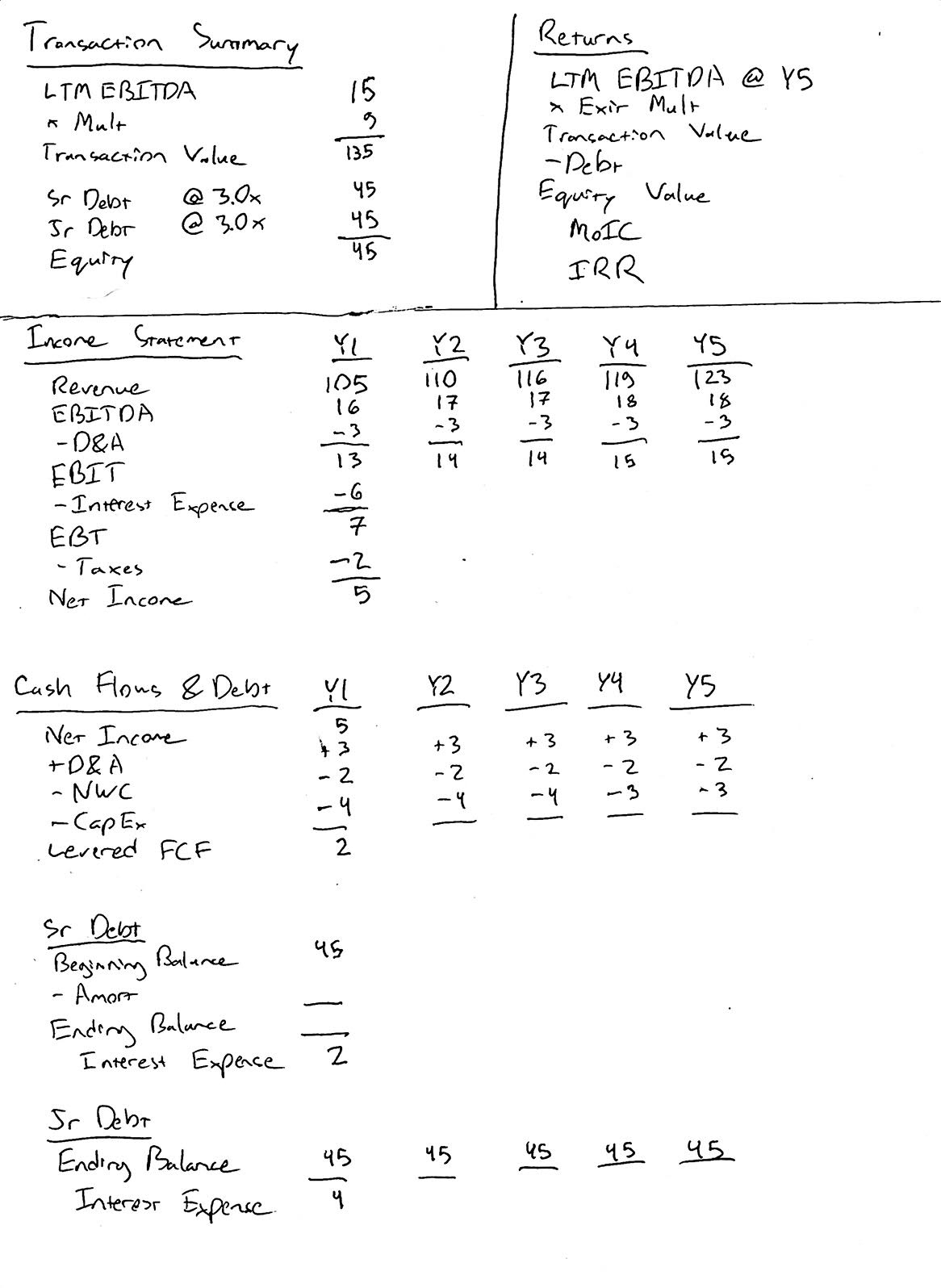### 6. Y1 Debt Paydown

Now that we’ve calculated the levered free cash flow (FCF) for Y1, we can calculate the senior debt amortization and ending balance.

We should pay down as much debt as possible, using all available cash flow (2mm). Therefore, our ending senior debt balance for Y1 is 43mm. And likewise, the beginning senior debt balance for Y2 is 43mm.

Here’s what your LBO should look like after this step: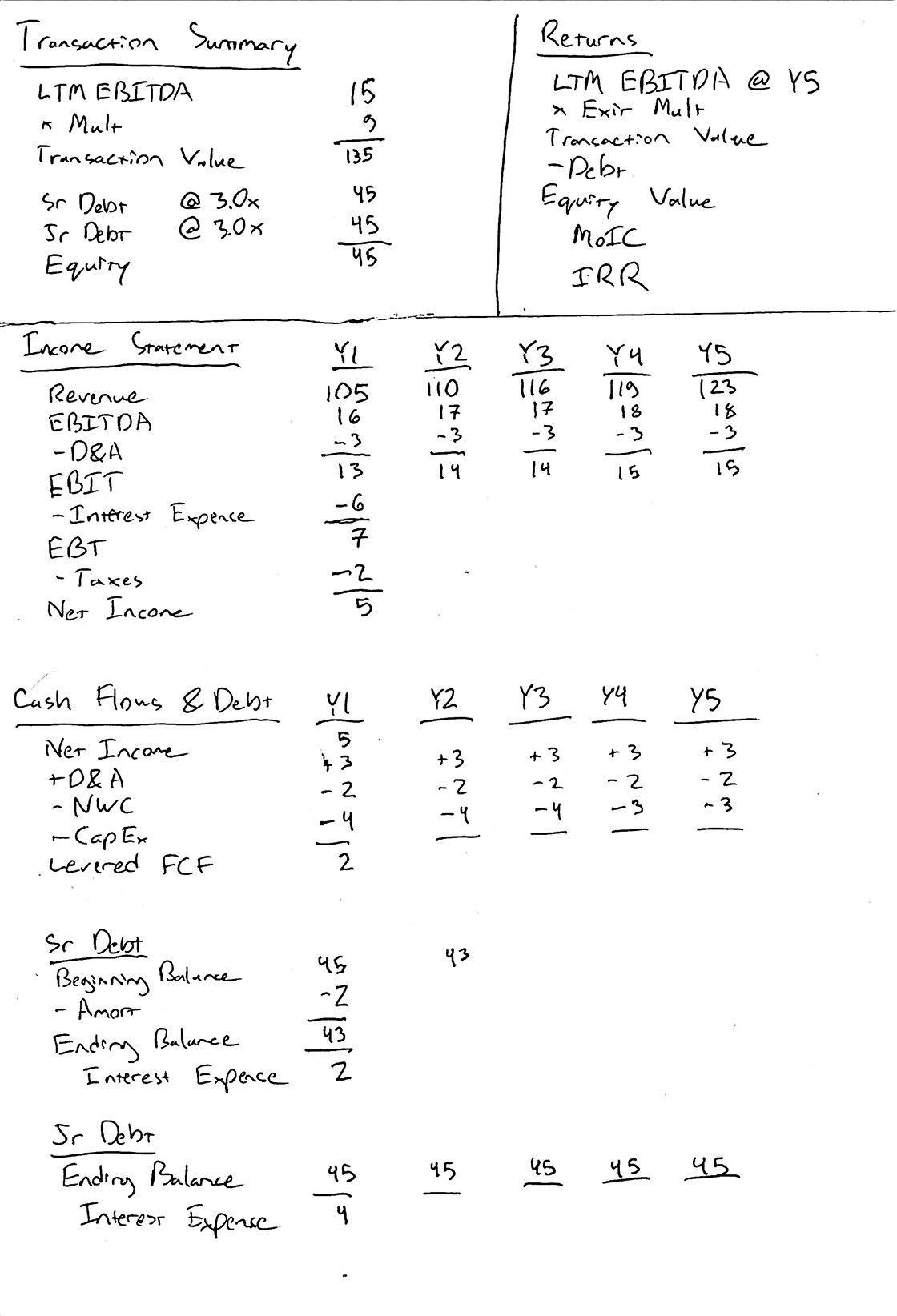### 7. Y2 - Y5 Interest Expense, Cash Flows & Debt Paydown

We’ve calculated the interest expense, levered FCF and debt paydown for Y1. Let’s fill out the remaining years following this pattern.

Here’s what your LBO should look like after this step: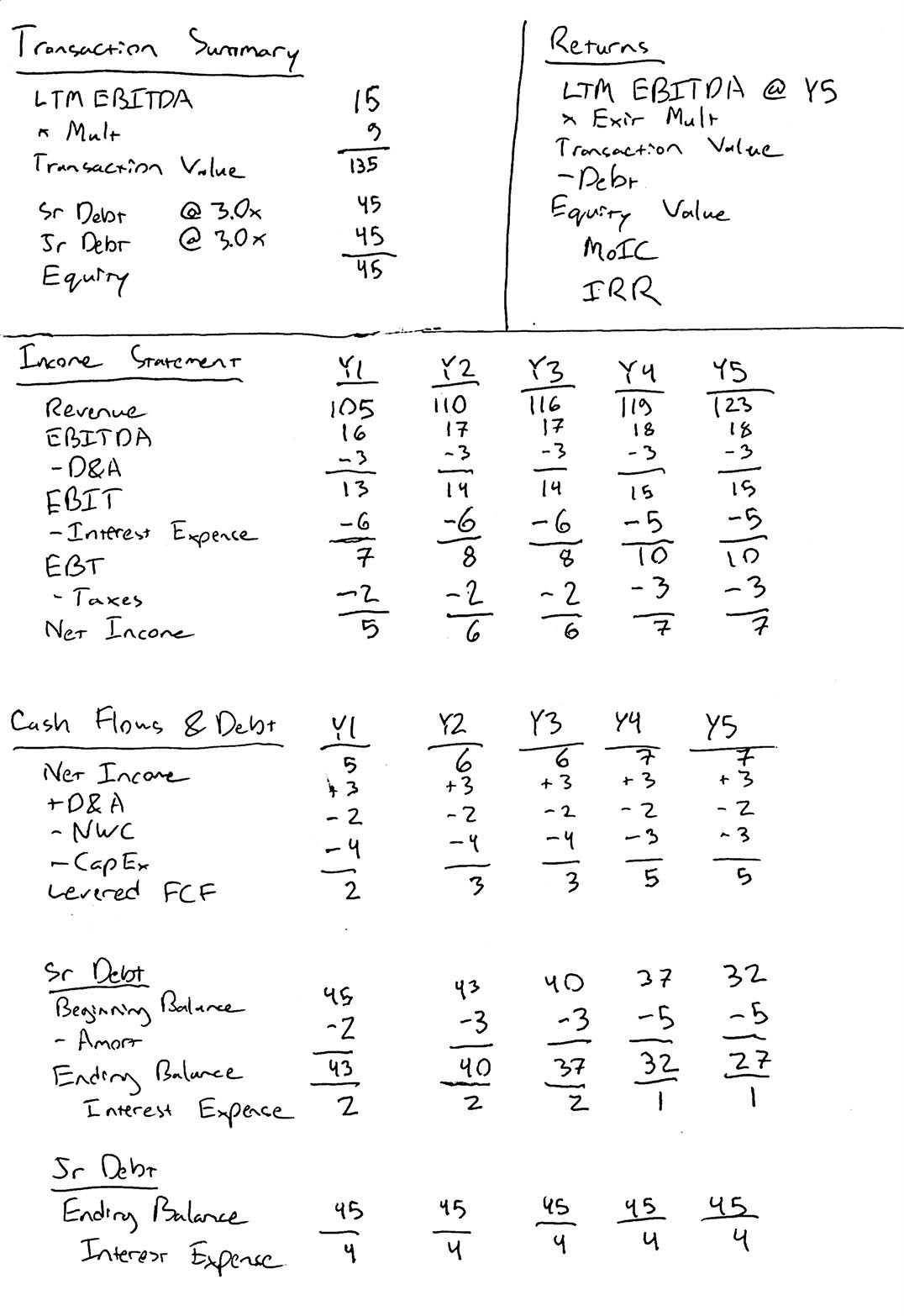### 8. Returns Summary

At this point, calculating the sponsor returns should be straightforward.

• Y5 LTM EBITDA is 18mm. The transaction value = LTM EBITDA (18mm) x 9.5 = 171mm.
• We have 72mm remaining debt (27mm senior debt + 45mm junior debt).
• Therefore, the sponsor equity value = 99mm, yielding a MoIC of 2.20x and a 17% IRR.

Here’s the completed paper LBO:## Bonus Question

You may have noticed that the returns above benefited from multiple expansion.

What would the sponsor returns have been without multiple expansion?

• Transaction Value = 18mm x 9 = 162mm.
• Equity Value = Transaction Value (162mm) - Debt (72mm) = 90mm
• MoIC = Equity Value (90mm) / Sponsor Equity (45mm) = 2.00x
• IRR = 15%

## Final Thoughts

Again, practice makes perfect. Once you can fly through this example under 10 minutes, you will be well prepared for any paper LBO. Sometimes interviewers include more manual calculations (e.g., D&A as percentage of revenue), but that should not increase the difficulty of the exercise. Most paper LBO exercises that you encounter will be simpler than this one.

## Next Steps

To learn more, check out our comprehensive (and free) private equity modeling course.

Also, here’s another practice paper LBO based on a real modeling test.